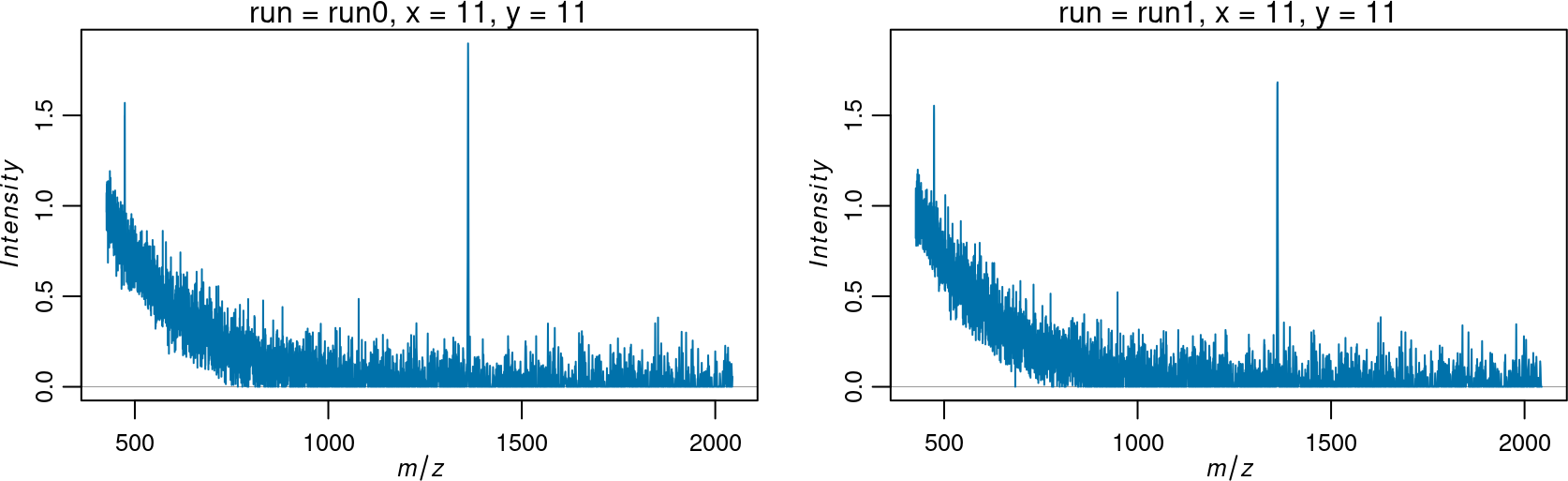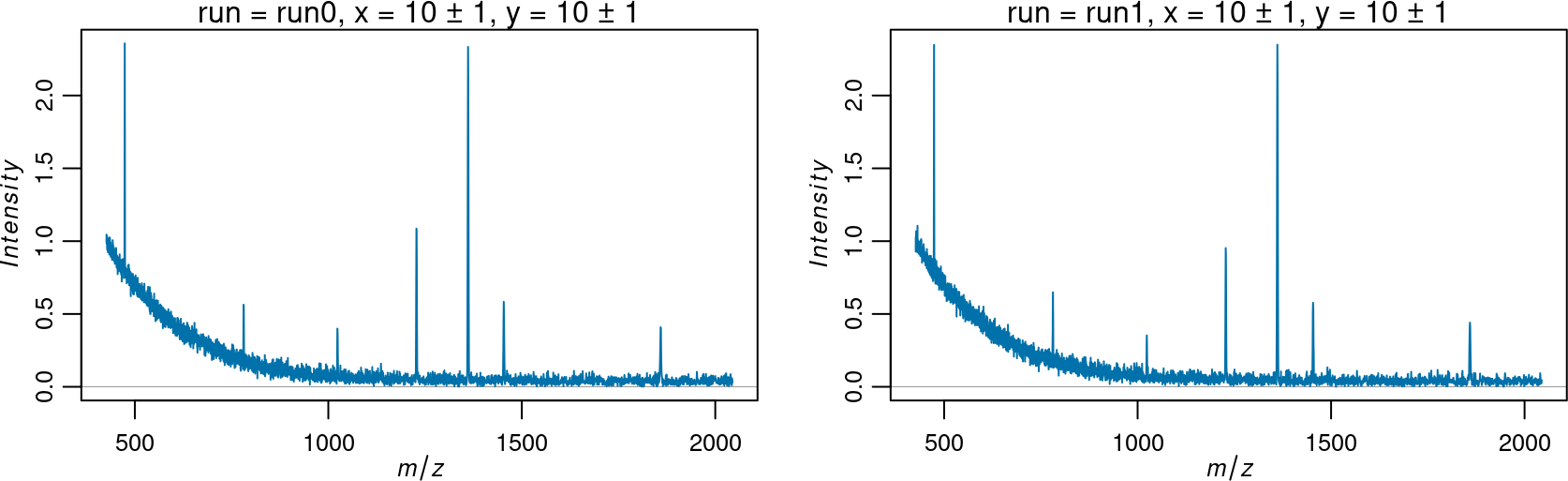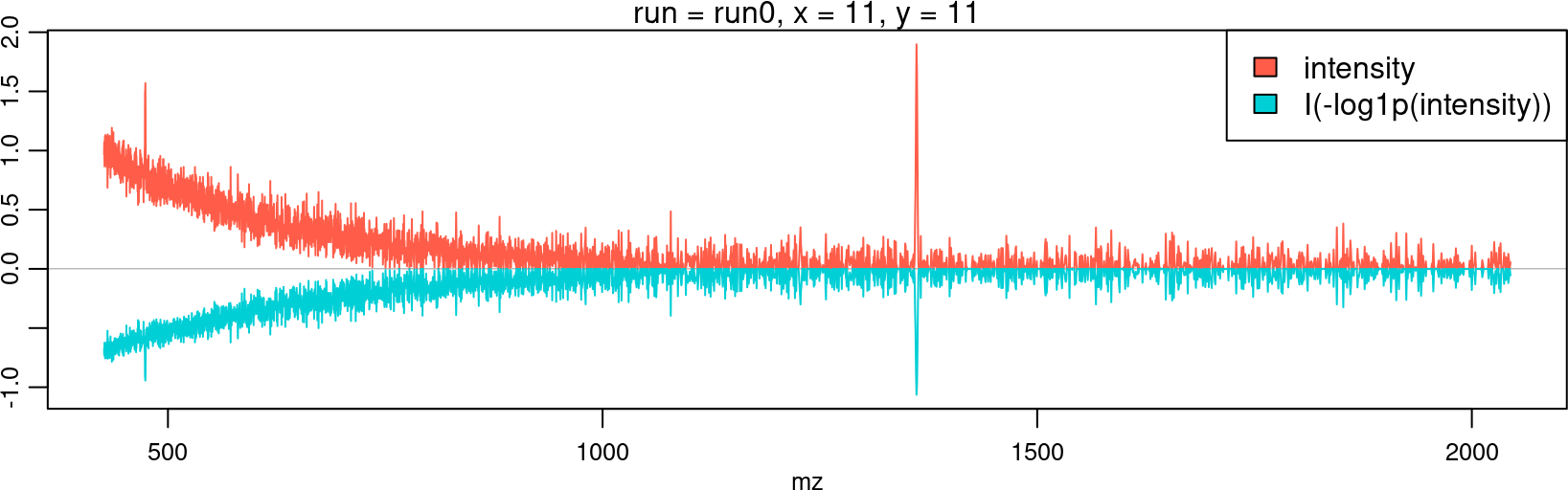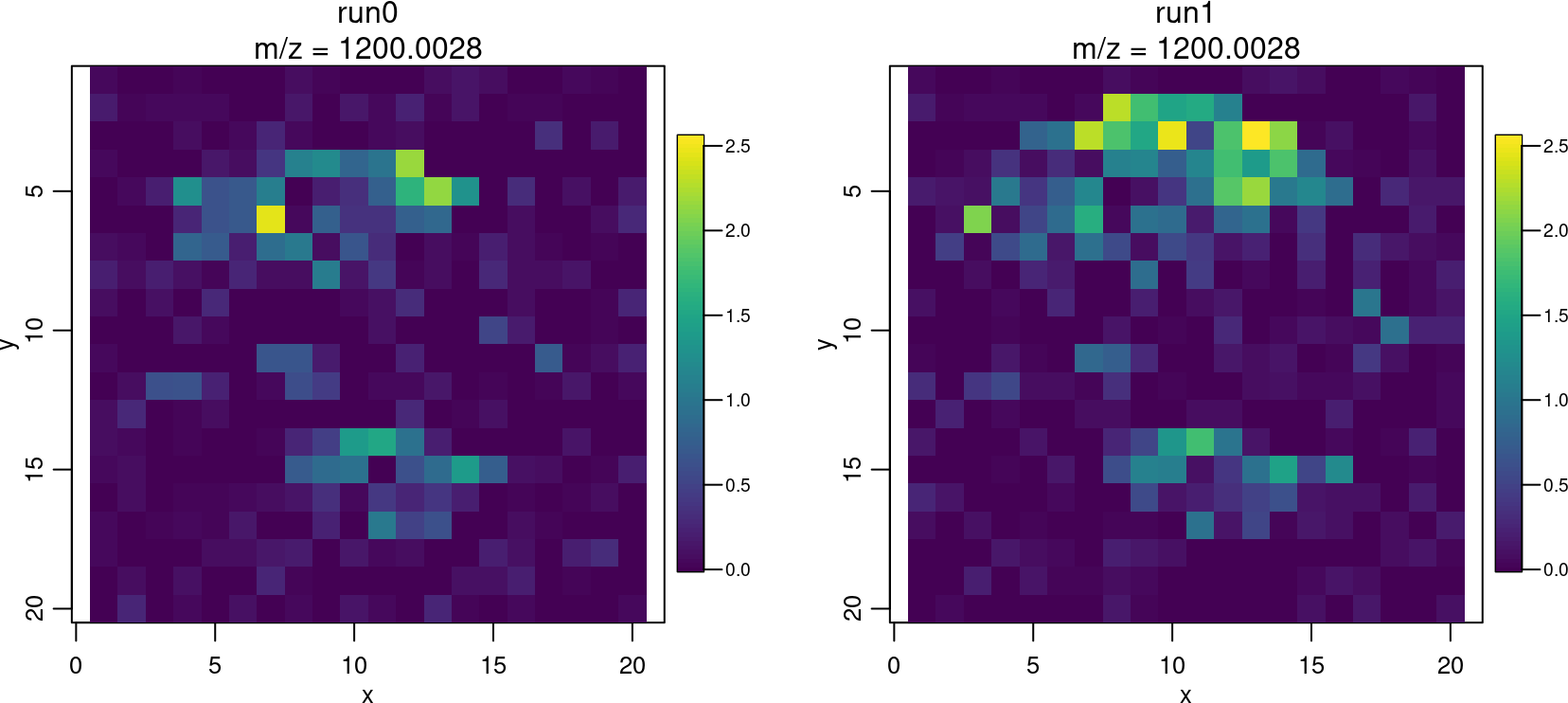# 1 Introduction

## 1.1 Cardinal 3

Cardinal 3 lays the groundwork for future improvements to the existing toolbox of pre-processing, visualization, and statistical methods for mass spectrometry (MS) imaging experiments. Cardinal has been updated to support matter 2, and legacy support has been dropped.

Despite minimal user-visible changes in Cardinal (at first), the entire matter package that provides the backend for Cardinal’s computing on larger-than-memory MS imaging datasets has been rewritten. This should provide more robust support for larger-than-memory computations, as well as greater flexibility in handling many data files in the future.

Further changes will be coming soon to Cardinal 3 in future point updates that are aimed to greatly improve the user experience and simplify the code that users need to write to process and analyze MS imaging data.

Major improvements from earlier versions are further described below.

## 1.2 Cardinal 2

Cardinal 2 provides new classes and methods for the manipulation, transformation, visualization, and analysis of imaging experiments–specifically MS imaging experiments.

MS imaging is a rapidly advancing field with consistent improvements in instrumentation for both MALDI and DESI imaging experiments. Both mass resolution and spatial resolution are steadily increasing, and MS imaging experiments grow increasingly complex.

The first version of Cardinal was written with certain assumptions about MS imaging data that are no longer true. For example, the basic assumption that the raw spectra can be fully loaded into memory is unreasonable for many MS imaging experiments today.

Cardinal 2 was re-written from the ground up to handle the evolving needs of high-resolution MS imaging experiments. Some advancements include:

• New imaging experiment classes such as `ImagingExperiment`, `SparseImagingExperiment`, and `MSImagingExperiment` provide better support for out-of-memory datasets and a more flexible representation of complex experiments

• New imaging metadata classes such as `PositionDataFrame` and `MassDataFrame` make it easier to manipulate experimental runs, pixel coordinates, and m/z-values by storing them as separate slots rather than ordinary columns

• New `plot()` and `image()` visualization methods that can handle non-gridded pixel coordinates and allow assigning the resulting plot (and data) to a variable for later re-plotting

• Support for writing imzML in addition to reading it; more options and support for importing out-of-memory imzML for both “continuous” and “processed” formats

• Data manipulation and summarization verbs such as `subset()`, `aggregate()`, and `summarizeFeatures()`, etc. for easier subsetting and summarization of imaging datasets

• Delayed pre-processing via a new `process()` method that allows queueing of delayed pre-processing methods such as `normalize()` and `peakPick()` for later execution

• Parallel processing support via the BiocParallel package for all pre-processing methods and any statistical analysis methods with a `BPPARAM` option

Classes from older versions of Cardinal should be coerced to their Cardinal 2 equivalents. For example, to return an updated `MSImageSet` object called `x`, use `as(x, "MSImagingExperiment")`.

# 2 Installation

Cardinal can be installed via the BiocManager package.

``````install.packages("BiocManager")
BiocManager::install("Cardinal")``````

The same function can be used to update Cardinal and other Bioconductor packages.

Once installed, Cardinal can be loaded with `library()`:

``library(Cardinal)``

# 3 Components of an `MSImagingExperiment`

In Cardinal, imaging experiment datasets are composed of multiple sets of metadata, in addition to the actual experimental data. These are (1) pixel metadata, (2) feature (\(m/z\)) metadata, (3) the actual imaging data, and (4) a class that holds all of these and represents the experiment as a whole.

`MSImagingExperiment` is a matrix-like container for complete MS imaging experiments, where the “rows” represent the mass features, and the columns represent the pixels. An `MSImagingExperiment` object contains the following components:

• `pixelData()` accesses the pixel information, stored in a `PositionDataFrame`

• `featureData()` accesses the feature information, stored in `MassDataFrame`

• `imageData()` accesses the spectral information, stored in a `ImageArrayList`

Unlike many software packages designed for analysis of MS imaging experiments, Cardinal is designed to work with multiple datasets simultaneously and can integrate all aspects of experimental design and metadata.

## 3.1 Example data

We will use `simulateImage()` to prepare an example dataset.

``````set.seed(2020)
mse <- simulateImage(preset=1, npeaks=10, nruns=2, baseline=1)
mse``````
``````## An object of class 'MSContinuousImagingExperiment'
##   <3919 feature, 800 pixel> imaging dataset
##     imageData(1): intensity
##     featureData(0):
##     pixelData(1): circle
##     run(2): run0 run1
##     raster dimensions: 20 x 20
##     coord(2): x = 1..20, y = 1..20
##     mass range:  426.5285 to 2044.4400
##     centroided: FALSE``````

## 3.2 Pixel metadata with `PositionDataFrame`

The `pixelData()` accessor extracts the pixel information for an `MSImagingExperiment`. The pixel data are stored in a `PositionDataFrame` object, which is a type of data frame that separately tracks pixel coordinates and experimental run information.

``pixelData(mse)``
``````## PositionDataFrame with 800 rows and 1 column
##        :run:   coord:x   coord:y    circle
##     <factor> <integer> <integer> <logical>
## 1       run0         1         1     FALSE
## 2       run0         2         1     FALSE
## 3       run0         3         1     FALSE
## 4       run0         4         1     FALSE
## 5       run0         5         1     FALSE
## ...      ...       ...       ...       ...
## 796     run1        16        20     FALSE
## 797     run1        17        20     FALSE
## 798     run1        18        20     FALSE
## 799     run1        19        20     FALSE
## 800     run1        20        20     FALSE``````

The `coord()` accessor specifically extracts the data frame of pixel coordinates.

``coord(mse)``
``````## DataFrame with 800 rows and 2 columns
##             x         y
##     <integer> <integer>
## 1           1         1
## 2           2         1
## 3           3         1
## 4           4         1
## 5           5         1
## ...       ...       ...
## 796        16        20
## 797        17        20
## 798        18        20
## 799        19        20
## 800        20        20``````

The `run()` accessor specifically extracts the vector of experimental runs.

``run(mse)[1:10]``
``````##   run0 run0 run0 run0 run0 run0 run0 run0 run0 run0
## Levels: run0 run1``````

## 3.3 Feature metadata with `MassDataFrame`

The `featureData()` accessor extracts the feature information for an `MSImagingExperiment`. The feature data are stored in a `MassDataFrame` object, which is a type of data frame that separately tracks the m/z-values associated with the mass spectral features.

``featureData(mse)``
``````## MassDataFrame with 3919 rows and 0 columns
##           :mz:
##      <numeric>
## 1      426.529
## 2      426.699
## 3      426.870
## 4      427.041
## 5      427.211
## ...        ...
## 3915   2041.17
## 3916   2041.99
## 3917   2042.81
## 3918   2043.62
## 3919   2044.44``````

The `mz()` accessor specifically extracts the vector of m/z-values.

``mz(mse)[1:10]``
``````##   426.5285 426.6991 426.8699 427.0406 427.2115 427.3824 427.5534 427.7245
##   427.8956 428.0668``````

## 3.4 Accessing spectra and image data

The `imageData()` accessor extracts the image data for an `MSImagingExperiment`. The data are stored in an `ImageArrayList`, which is a list of matrix-like objects.

It is possible to store multiple matrices of intensities in this list. Typically, only the first entry will be used by pre-processing and analysis methods.

``imageData(mse)``
``````## MSContinuousImagingSpectraList of length 1
##  names(1):    intensity
##  class(1):       matrix
##    dim(1): <3919 x 800>
##    mem(1):     25.08 MB``````

Entries in this list can be extracted by name with `iData()`.

``iData(mse, "intensity")``

The `spectra()` accessor is a shortcut for accessing the first data matrix.

``spectra(mse)[1:5, 1:5]``
``````##           [,1]      [,2]      [,3]      [,4]      [,5]
## [1,] 0.9295940 0.9779923 0.9415157 0.9115036 0.8960595
## [2,] 1.0087009 1.3108664 1.0928983 1.0243944 1.0706272
## [3,] 1.0578001 1.0625834 1.2407371 0.9319758 0.8822412
## [4,] 0.8949165 1.1568158 0.9250994 0.9499621 1.0127282
## [5,] 1.0660395 1.0123048 1.0291570 0.8999156 1.1126816``````

Rows of these matrices correspond to mass features. Columns correspond to pixels. In other words, each column is a mass spectrum, and each row is a flattened vector of images.

## 3.5 Specialized classes

In order to specialize to the needs of different datasets, Cardinal provides specialized versions of `MSImagingExperiment` that reflect different spectra storage strategies.

### 3.5.1 “Continuous” MS imaging experiments

`MSContinuousImagingExperiment` is a specialization that enforces the data matrices be stored as dense, column-major matrices. These include R’s native `matrix` and `matter_matc` objects from the matter package.

``mse``
``````## An object of class 'MSContinuousImagingExperiment'
##   <3919 feature, 800 pixel> imaging dataset
##     imageData(1): intensity
##     featureData(0):
##     pixelData(1): circle
##     run(2): run0 run1
##     raster dimensions: 20 x 20
##     coord(2): x = 1..20, y = 1..20
##     mass range:  426.5285 to 2044.4400
##     centroided: FALSE``````
``imageData(mse)``
``````## MSContinuousImagingSpectraList of length 1
##  names(1):    intensity
##  class(1):       matrix
##    dim(1): <3919 x 800>
##    mem(1):     25.08 MB``````

### 3.5.2 “Processed” MS imaging experiments

`MSProcessedImagingExperiment` is a specialization that enforces the data matrices be stored as sparse, column-major matrices. These include `sparse_matc` objects from the matter package. This specialization is useful for processed data.

``````set.seed(2020)
mse2 <- simulateImage(preset=3, npeaks=10, nruns=2)
mse3 <- as(mse2, "MSProcessedImagingExperiment")
mse3``````
``````## An object of class 'MSProcessedImagingExperiment'
##   <3919 feature, 800 pixel> imaging dataset
##     imageData(1): intensity
##     featureData(0):
##     pixelData(3): square1 square2 circle
##     run(2): run0 run1
##     raster dimensions: 20 x 20
##     coord(2): x = 1..20, y = 1..20
##     mass range:  426.5285 to 2044.4400
##     centroided: FALSE``````
``imageData(mse3)``
``````## MSProcessedImagingSpectraList of length 1
##  names(1):    intensity
##  class(1):   sparse_mat
##    dim(1): <3919 x 800>
##    mem(1):     50.21 MB``````

Because the data is stored sparsely, spectra from `MSProcessedImagingExperiment` objects are binned on-the-fly to the m/z-values specified by `mz()`.

The resolution of the binning can be accessed by `resolution()`.

``resolution(mse3)``
``````## ppm
## 400``````

The resolution can be set to change how the binning is performed.

``resolution(mse3) <- c(ppm=200)``

Changing the binned mass resolution will typically change the effective dimensions of the experiment.

``dim(mse2)``
``````## Features   Pixels
##     3919      800``````
``dim(mse3)``
``````## Features   Pixels
##     7837      800``````

The effective m/z-values are also updated to reflect the new bins.

``mz(mse2)[1:10]``
``````##   426.5285 426.6991 426.8699 427.0406 427.2115 427.3824 427.5534 427.7245
##   427.8956 428.0668``````
``mz(mse3)[1:10]``
``````##   426.5285 426.6138 426.6991 426.7845 426.8699 426.9552 427.0406 427.1261
##   427.2115 427.2969``````

Note that the underlying data will remain unchanged, but the binned values for the intensities will be different.

# 4 Data import and export

Cardinal 2 natively supports reading and writing imzML (both “continuous” and “processed” versions) and Analyze 7.5 formats via the `readMSIData()` and `writeMSIData()` functions.

We will focus on imzML.

The imzML format is an open standard designed specifically for interchange of mass spectrometry imaging datasets. Many other formats can be converted to imzML with the help of free applications available online at .

Let’s create a small image to demonstrate data import/export.

``````set.seed(2020)
tiny <- simulateImage(preset=1, from=500, to=600, dim=c(3,3))
tiny``````
``````## An object of class 'MSContinuousImagingExperiment'
##   <456 feature, 9 pixel> imaging dataset
##     imageData(1): intensity
##     featureData(0):
##     pixelData(1): circle
##     run(1): run0
##     raster dimensions: 3 x 3
##     coord(2): x = 1..3, y = 1..3
##     mass range: 500.0000 to 599.8071
##     centroided: FALSE``````

We’ll also create a “processed” version for writing “processed” imzML.

``````tiny2 <- as(tiny, "MSProcessedImagingExperiment")
tiny2``````
``````## An object of class 'MSProcessedImagingExperiment'
##   <456 feature, 9 pixel> imaging dataset
##     imageData(1): intensity
##     featureData(0):
##     pixelData(1): circle
##     run(1): run0
##     raster dimensions: 3 x 3
##     coord(2): x = 1..3, y = 1..3
##     mass range: 500.0000 to 599.8071
##     centroided: FALSE``````

Note that despite the name, the only difference between “continuous” and “processed” imzML is how the data are stored, rather than what processing has been applied to the data. “Continuous” imzML stores spectra densely, with each spectrum sharing the same m/z-values. “Processed” imzML stores spectra sparsely, and each spectrum can have its own distinct m/z-values.

## 4.1 Writing imzML

Use `writeMSIData()` to write datasets to imzML and Analyze formats.

### 4.1.1 Writing “continuous” imzML

Internally, `writeMSIData()` will call either `writeImzML()` or `writeAnalyze()` depending on the value of `outformat`. The default is `outformat="imzML"`.

``````path <- tempfile()
writeMSIData(tiny, file=path, outformat="imzML")``````

Two files are produced with extensions “.imzML” and “.ibd”. The former contains an XML description of the dataset, and the latter contains the actual intensity data.

``##  "file351679351b7bd2.ibd"   "file351679351b7bd2.imzML"``

Because `tiny` is a `MSContinuousImagingExperiment`, it is written as “continuous” imzML.

``````mzml <- readLines(paste0(path, ".imzML"))
grep("continuous", mzml, value=TRUE)``````
``##  "\t\t\t<cvParam cvRef=\"IMS\" accession=\"IMS:1000030\" name=\"continuous\" value=\"\" />"``

### 4.1.2 Writing “processed” imzML

We can also write “processed” imzML if we export a `MSProcessedImagingExperiment` file.

``````path2 <- tempfile()
writeMSIData(tiny2, file=path2, outformat="imzML")``````
``````mzml2 <- readLines(paste0(path2, ".imzML"))
grep("processed", mzml2, value=TRUE)``````
``##  "\t\t\t<cvParam cvRef=\"IMS\" accession=\"IMS:1000031\" name=\"processed\" value=\"\" />"``

### 4.1.3 Writing datasets with multiple runs

If an experiment contains multiple runs, then each run will be written to a separate imzML file.

``````set.seed(2020)
tiny3 <- simulateImage(preset=1, from=500, to=600, dim=c(3,3), nruns=2)
runNames(tiny3)``````
``##  "run0" "run1"``
``````path3 <- tempfile()
writeMSIData(tiny3, file=path3, outformat="imzML")``````
``````##  "file3516797783d077-run0.ibd"   "file3516797783d077-run0.imzML"
##  "file3516797783d077-run1.ibd"   "file3516797783d077-run1.imzML"``````

Use `readMSIData()` to read datasets in imzML or Analyze formats.

Internally, `readMSIData()` will guess the format based on the file extension (which must be included) and call either `readImzML()` or `readAnalyze()`.

``````path_in <- paste0(path, ".imzML")
tiny_in``````
``````## An object of class 'MSContinuousImagingExperiment'
##   <456 feature, 9 pixel> imaging dataset
##     imageData(1): intensity
##     featureData(0):
##     pixelData(0):
##     run(1): file351679351b7bd2
##     raster dimensions: 3 x 3
##     coord(2): x = 1..3, y = 1..3
##     mass range: 500.0000 to 599.8071
##     centroided: FALSE``````

The `attach.only` argument is used to specify that the intensity data should not be loaded into memory, but instead attached as a file-based matrix using the matter package.

Starting in Cardinal 2, the default is `attach.only=TRUE`. This is more memory-efficient, but some methods may be more time-consuming due to the file I/O.

``imageData(tiny_in)``
``````## MSContinuousImagingSpectraList of length 1
##  names(1):  intensity
##  class(1): matter_mat
##    dim(1):  <456 x 9>
##    mem(1):    6.98 KB``````
``spectra(tiny_in)``
``````## <456 row x 9 col> matter_mat :: out-of-memory double matrix
##      Spectrum=1 Spectrum=2 Spectrum=3 Spectrum=4 Spectrum=5 Spectrum=6 ...
## [1,] 0.00000000 0.04087833 0.00000000 0.00000000 0.13038468 0.03781159 ...
## [2,] 0.00000000 0.16240965 0.00000000 0.16388728 0.00000000 0.00000000 ...
## [3,] 0.00000000 0.00000000 0.03591078 0.00000000 0.00000000 0.09754688 ...
## [4,] 0.18744168 0.04057066 0.08853593 0.00000000 0.01664007 0.03274257 ...
## [5,] 0.00000000 0.19307238 0.00000000 0.00000000 0.00000000 0.00000000 ...
## [6,] 0.30000976 0.00000000 0.08789141 0.01251065 0.07945876 0.08794949 ...
## ...         ...        ...        ...        ...        ...        ... ...
## (6.98 KB real | 16.42 KB virtual)``````

An out-of-memory matter matrix can be subsetted like an ordinary R matrix. The data values are only read from file and loaded into memory when they are requested (via subsetting).

``spectra(tiny_in)[1:5, 1:5]``
``````##      Spectrum=1 Spectrum=2 Spectrum=3 Spectrum=4 Spectrum=5
## [1,]  0.0000000 0.04087833 0.00000000  0.0000000 0.13038468
## [2,]  0.0000000 0.16240965 0.00000000  0.1638873 0.00000000
## [3,]  0.0000000 0.00000000 0.03591078  0.0000000 0.00000000
## [4,]  0.1874417 0.04057066 0.08853593  0.0000000 0.01664007
## [5,]  0.0000000 0.19307238 0.00000000  0.0000000 0.00000000``````

If loading the data fully into memory is desired, either set `attach.only=FALSE` when reading the data, or use `as.matrix()` on the intensity matrix.

``````spectra(tiny_in) <- as.matrix(spectra(tiny_in))
imageData(tiny_in)``````
``````## MSContinuousImagingSpectraList of length 1
##  names(1): intensity
##  class(1):    matrix
##    dim(1): <456 x 9>
##    mem(1):  33.98 KB``````

Using `collect()` on the `MSImagingExperiment` will also load all data into memory.

``tiny_in <- collect(tiny_in)``

For “processed” imzML files, the spectra must be binned to common m/z-values. By default, `readImzML()` will detect the mass range from the data. This requires reading a large proportion of data from the file, even if `attach.only=TRUE`.

``````path2_in <- paste0(path2, ".imzML")
tiny2_in``````
``````## An object of class 'MSProcessedImagingExperiment'
##   <456 feature, 9 pixel> imaging dataset
##     imageData(1): intensity
##     featureData(0):
##     pixelData(0):
##     run(1): file351679b774db5
##     raster dimensions: 3 x 3
##     coord(2): x = 1..3, y = 1..3
##     mass range: 500.0000 to 599.8071
##     centroided: FALSE``````

If known, it can be much more efficient to specify `mass.range` when importing “processed” imzML data. This can also be used to pre-filter the data to a smaller mass range.

The size of the m/z bins can be changed with the `resolution` argument.

``````tiny2_in <- readMSIData(path2_in, mass.range=c(510,590),
resolution=100, units="ppm")
tiny2_in``````
``````## An object of class 'MSProcessedImagingExperiment'
##   <1458 feature, 9 pixel> imaging dataset
##     imageData(1): intensity
##     featureData(0):
##     pixelData(0):
##     run(1): file351679b774db5
##     raster dimensions: 3 x 3
##     coord(2): x = 1..3, y = 1..3
##     mass range: 510.000 to 589.993
##     centroided: FALSE``````

The resolution for the m/z bins can be changed after importing the data by setting the `resolution()` of the dataset.

``resolution(tiny2_in) <- c(ppm=400)``

## 4.3 Building from scratch

While importing formats besides imzML and Analyze are not explicitly supported by Cardinal, if the data can be read into R, it is easy to construct a `MSImagingExperiment` object from its components.

``````set.seed(2020)
s <- simulateSpectrum(n=9, peaks=10, from=500, to=600)

coord <- expand.grid(x=1:3, y=1:3)
run <- factor(rep("run0", nrow(coord)))

fdata <- MassDataFrame(mz=s\$mz)
pdata <- PositionDataFrame(run=run, coord=coord)

out <- MSImagingExperiment(imageData=s\$intensity,
featureData=fdata,
pixelData=pdata)
out``````
``````## An object of class 'MSContinuousImagingExperiment'
##   <456 feature, 9 pixel> imaging dataset
##     imageData(1): intensity
##     featureData(0):
##     pixelData(0):
##     run(1): run0
##     raster dimensions: 3 x 3
##     coord(2): x = 1..3, y = 1..3
##     mass range: 500.0000 to 599.8071
##     centroided: FALSE``````

For loading data into R, `read.csv()` and `read.table()` can be used to read CSV and tab-delimited text files, respectively.

Likewise, `write.csv()` and `write.table()` can be used to write pixel metadata and feature metadata after coercing them to an ordinary R `data.frame` with `as.data.frame()`.

Use `saveRDS()` and `readRDS()` to save and read and entire R object such as a `MSImagingExperiment`. Note that if intensity data is to be saved as well, it should be pulled into memory and coerced to an R matrix with `as.matrix()` first.

# 5 Visualization

Visualization of mass spectra and molecular ion images is vital for exploratory analysis of MS imaging experiments. Cardinal provides a `plot()` method for plotting mass spectra and an `image()` method for plotting ion images.

Cardinal 2 provides some useful visualization updates compared to previous versions:

• A new default color scale (viridis) for images that doesn’t use the flawed rainbow color scheme

• Non-gridded pixel coordinates are allowed, and plotting of non-rastered image data is better supported

• The new `plot()` and `image()` methods return values that can be assigned to a variable for later re-plotting

## 5.1 Visualizing mass spectra with `plot()`

Use `plot()` to plot mass spectra. Either `pixel` or `coord` can be specified to determine which mass spectra should be plotted.

``plot(mse, pixel=c(211, 611))``The `plusminus` argument can be used to specify that multiple spectra should be averaged and plotted together.

``plot(mse, coord=list(x=10, y=10), plusminus=1, fun=mean)``A formula can be specified to further customize mass spectra plotting. The LHS of the formula should specify one or more elements of `imageData()` and the RHS of the formula should be a variable in `featureData()`.

``plot(mse, intensity + I(-log1p(intensity)) ~ mz, pixel=211, superpose=TRUE)``## 5.2 Visualizing images with `image()`

Use `image()` to plot ion images. Either `feature` or `mz` can be specified to determine which mass features should be plotted.

``image(mse, mz=1200)``The `plusminus` argument can be used to specify that multiple mass features should be averaged and plotted together.

``image(mse, mz=1227, plusminus=0.5, fun=mean)``